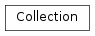# mvpa2.datasets.cosmo.Collection¶class `mvpa2.datasets.cosmo.``Collection`(items=None)

Container of some Collectables.

Methods

`clear`(() -> None.  Remove all items from D.)
`copy`([deep, a, memo]) Create a copy of a collection.
`fromkeys`(...) v defaults to None.
`get`((k[,d]) -> D[k] if k in D, ...)
`has_key`((k) -> True if D has a key k, else False)
`items`(() -> list of D’s (key, value) pairs, ...)
`iteritems`(() -> an iterator over the (key, ...)
`iterkeys`(() -> an iterator over the keys of D)
`itervalues`(...)
`keys`(() -> list of D’s keys)
`pop`((k[,d]) -> v, ...) If key is not found, d is returned if given, otherwise KeyError is raised
`popitem`(() -> (k, v), ...) 2-tuple; but raise KeyError if D is empty.
`setdefault`((k[,d]) -> D.get(k,d), ...)
`update`(source[, copyvalues, memo])
`values`(() -> list of D’s values)
`viewitems`(...)
`viewkeys`(...)
`viewvalues`(...)
Parameters: items : all types accepted by update()

Methods

`clear`(() -> None.  Remove all items from D.)
`copy`([deep, a, memo]) Create a copy of a collection.
`fromkeys`(...) v defaults to None.
`get`((k[,d]) -> D[k] if k in D, ...)
`has_key`((k) -> True if D has a key k, else False)
`items`(() -> list of D’s (key, value) pairs, ...)
`iteritems`(() -> an iterator over the (key, ...)
`iterkeys`(() -> an iterator over the keys of D)
`itervalues`(...)
`keys`(() -> list of D’s keys)
`pop`((k[,d]) -> v, ...) If key is not found, d is returned if given, otherwise KeyError is raised
`popitem`(() -> (k, v), ...) 2-tuple; but raise KeyError if D is empty.
`setdefault`((k[,d]) -> D.get(k,d), ...)
`update`(source[, copyvalues, memo])
`values`(() -> list of D’s values)
`viewitems`(...)
`viewkeys`(...)
`viewvalues`(...)
`copy`(deep=True, a=None, memo=None)

Create a copy of a collection.

By default this is going to return a deep copy of the collection, hence no data would be shared between the original dataset and its copy.

Parameters: deep : boolean, optional If False, a shallow copy of the collection is return instead. The copy contains only views of the values. a : list or None List of attributes to include in the copy of the dataset. If `None` all attributes are considered. If an empty list is given, all attributes are stripped from the copy. memo : dict Developers only: This argument is only useful if copy() is called inside the __deepcopy__() method and refers to the dict-argument `memo` in the Python documentation.
`update`(source, copyvalues=None, memo=None)
Parameters: source : list, Collection, dict copyvalues : None, shallow, deep If None, values will simply be bound to the collection items’ values thus sharing the same instance. ‘shallow’ and ‘deep’ copies use ‘copy’ and ‘deepcopy’ correspondingly. memo : dict Developers only: This argument is only useful if copy() is called inside the __deepcopy__() method and refers to the dict-argument `memo` in the Python documentation.